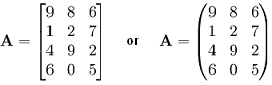HOME MATH DICTIONARY DOWNLOAD FEEDBACK DISCLAIMER
 Question: What is an Order of a Matrix ? Answer: A matrix is a rectangular array of numbers. The size and shape of the array is given by the number of rows and columns it contains, called its order. So a matrix with 3 rows and 2 columns is described as having order 3 by 2. This is not the same as a matrix of order 2 by 3, which has 2 rows and 3 columns.is a 4 x 3 matrix. The element a2,3 or A[2,3] is 7. In terms of the mathematical definition given above, this matrix is a function A : {1, 2, 3, 4} x {1, 2, 3} __> R